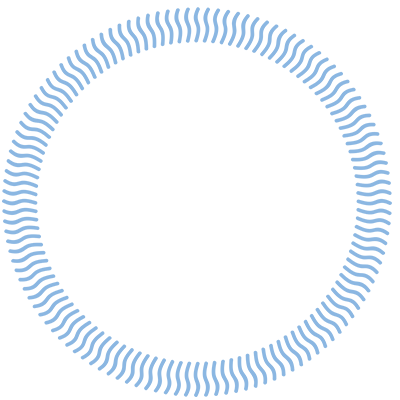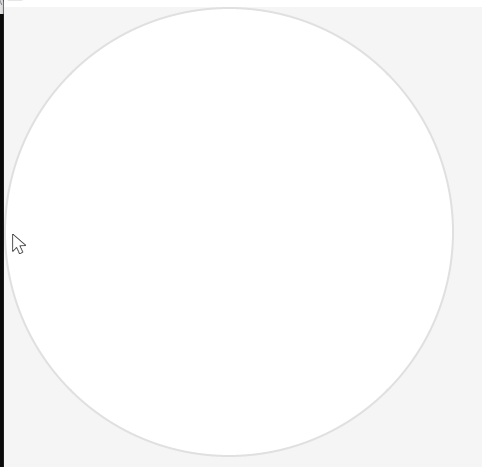# How to use lv_meter to realize the arc progress of gradient

How to use lv_meter to realize the arc progress of gradient.
Just like the picture.It’s not supported now on the arcs of the meter. Normal `lv_arc` supports it via the `arc_img_src` property. In `draw_arcs` in `lv_meter.c` you can set `arc_dsc.img_src = my_image_src`. This way LVGL will clip the arc from that image.

Of course, we can have improve this part and add a proper API for this.

OK.Thanks for your help.

I revised it according to your reply，but there is a new problem:I want to show this arc picture(the 1st picture) in meter,when i set the value less than 85,there is nothing in meter(like the 2nd picture).But when i set the value more than 85,the picture is normal show(like the 3rd picture).Here is my code:(1)test code:
void lv_example_meter_test(void)
{
meter = lv_meter_create(lv_scr_act());
lv_obj_align(meter,LV_ALIGN_TOP_LEFT,0,0);
lv_obj_set_size(meter, 450, 450);

``````/*Remove the circle from the middle*/
lv_obj_remove_style(meter, NULL, LV_PART_INDICATOR);

/*Add a scale first*/
lv_meter_scale_t * scale = lv_meter_add_scale(meter);
lv_meter_set_scale_range(meter, scale, 0, 100, 360, 90);
lv_meter_set_scale_ticks(meter, scale, 0, 0, 0, lv_palette_main(LV_PALETTE_RED));

/*Add a three arc indicator*/
lv_meter_indicator_t * indic = lv_meter_add_arc_with_img_src(meter, scale, 50, PIC_ABS_PATH("other/pirture.png"), 0);
lv_meter_set_indicator_end_value(meter,indic, 85);
``````

}

lv_meter_indicator_t * lv_meter_add_arc_with_img_src(lv_obj_t * obj, lv_meter_scale_t * scale, uint16_t width, void *src,
int16_t r_mod)
{
LV_ASSERT_OBJ(obj, MY_CLASS);
lv_meter_t * meter = (lv_meter_t *)obj;
lv_meter_indicator_t * indic = _lv_ll_ins_head(&meter->indicator_ll);
LV_ASSERT_MALLOC(indic);
lv_memset_00(indic, sizeof(lv_meter_indicator_t));
indic->scale = scale;
indic->opa = LV_OPA_COVER;

``````indic->type = LV_METER_INDICATOR_TYPE_ARC;
indic->type_data.arc.width = width;
indic->type_data.arc.src = src;
indic->type_data.arc.r_mod = r_mod;

lv_obj_invalidate(obj);
return indic;
``````

}

(3)draw_arcs function code
I add two lines of code in this function:
/*****************************
if(indic->type_data.arc.src){
part_draw_dsc.arc_dsc->img_src = indic->type_data.arc.src;
}
*****************************/

static void draw_arcs(lv_obj_t * obj, lv_draw_ctx_t * draw_ctx, const lv_area_t * scale_area)
{
lv_meter_t * meter = (lv_meter_t *)obj;

``````lv_draw_arc_dsc_t arc_dsc;
lv_draw_arc_dsc_init(&arc_dsc);
arc_dsc.rounded = lv_obj_get_style_arc_rounded(obj, LV_PART_ITEMS);

lv_coord_t r_out = lv_area_get_width(scale_area) / 2 ;
lv_point_t scale_center;
scale_center.x = scale_area->x1 + r_out;
scale_center.y = scale_area->y1 + r_out;

lv_opa_t opa_main = lv_obj_get_style_opa(obj, LV_PART_MAIN);
lv_meter_indicator_t * indic;

lv_obj_draw_part_dsc_t part_draw_dsc;
lv_obj_draw_dsc_init(&part_draw_dsc, draw_ctx);
part_draw_dsc.arc_dsc = &arc_dsc;
part_draw_dsc.part = LV_PART_INDICATOR;
part_draw_dsc.class_p = MY_CLASS;
part_draw_dsc.type = LV_METER_DRAW_PART_ARC;

if(indic->type != LV_METER_INDICATOR_TYPE_ARC) continue;

arc_dsc.color = indic->type_data.arc.color;
arc_dsc.width = indic->type_data.arc.width;
arc_dsc.opa = indic->opa > LV_OPA_MAX ? opa_main : (opa_main * indic->opa) >> 8;

lv_meter_scale_t * scale = indic->scale;

int32_t start_angle = lv_map(indic->start_value, scale->min, scale->max, scale->rotation,
scale->rotation + scale->angle_range);
int32_t end_angle = lv_map(indic->end_value, scale->min, scale->max, scale->rotation,
scale->rotation + scale->angle_range);

**if(indic->type_data.arc.src){**
``````

** part_draw_dsc.arc_dsc->img_src = indic->type_data.arc.src;**
** }**

``````    part_draw_dsc.radius = r_out + indic->type_data.arc.r_mod;
part_draw_dsc.sub_part_ptr = indic;
part_draw_dsc.p1 = &scale_center;

lv_event_send(obj, LV_EVENT_DRAW_PART_BEGIN, &part_draw_dsc);
lv_draw_arc(draw_ctx, &arc_dsc, &scale_center, part_draw_dsc.radius, start_angle, end_angle);
lv_event_send(obj, LV_EVENT_DRAW_PART_END, &part_draw_dsc);
}
``````

}

Adding `lv_meter_add_arc_with_img_src` sounds good. Can you send a pull request?
It’d make also easier to test the mentioned issue.

Sorry, I don’t understand. What should I do？

PR stands for Pull Request (i.e. a patch for LVGL). You acn create one as described here: Pull requests

Did you find a solution for this? I am having the same problem. I found that when the value is less than 85 it is drawing the image centered on the indicator. I discovered this by using a small test image. I have screenshots on How do I set the image source to mask out an arc on a complex/gradient image?